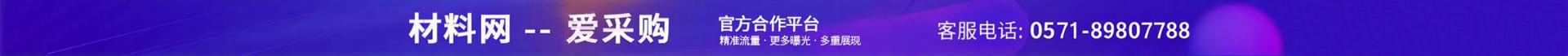•e="2796" et="1">

pe="381914">

e="915" et="4">

e="1563" et="2">

e="5752" et="5">